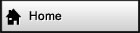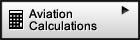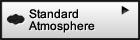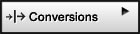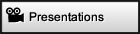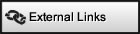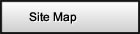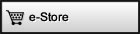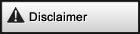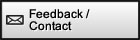If you would like to share,   e-mail or bookmark this   page, the tools below   are available for your   convenience.Surf with confidence!   Site has been tested by:Norton™ Safe WebMcAfee SiteAdvisor ®1976 Standard Atmosphere Properties Complete standard atmosphere calculations: All 28 functions as outlined by the standard1976 Standard Atmosphere Properties (0-86 km) Show additional decimal point
Variables
 Geometric Altitude (Z) [m] [ft] [sm] Geopotential Altitude (H) [m] [ft] [sm] Lapse rate (λ or LM) -0.0065 0.0000 0.0010 0.0028 0.0000 -0.0028 -0.0020          [K/m]    -0.01170   0.00000   0.00180   0.00504   0.00000  -0.00504  -0.00360    [oF/m] -1.98120   0.00000   0.30480   0.85344   0.00000  -0.85344  -0.60960   [oC/1000 ft]  -3.5662   0.0000   0.54864   1.5362   0.0000  -1.5362  -1.0973   [oF/1000 ft] Temperature Ratio (θ) Molecular Temperature (TM) [K]   [oR] [oC] [oF] Kinetic Temperature (T) [K]   [oR] [oC] [oF] Mean Molec. Wt. Ratio (M/M0) Mean Molecular Weight (M) [kg/kmol] or [lb/lbmol] Pressure Ratio (δ) Pressure (P) [Pa] or  [N/m2]   [hPa] or [mbar]   [lbf/ft2] [in Hg] [mmHg] or [torr] [lbf/in2] Density Ratio (σ) Density (ρ) [kg/m3] [slug/ft3] Gravitational Accel. Ratio (g/g0) Gravitational Acceleration (g) [m/s2] [ft/s2] Dynamic Viscosity Ratio (μ/μ0) Dynamic Viscosity (μ) [kg/(m·s)] [slug/(ft·s)] Kinematic Viscosity Ratio (η/η0) Kinematic Viscosity (η) [m2/s] [ft2/s] Speed of Sound Ratio (CS/CS0) Speed of Sound (CS) [m/s] [ft/s] [Kts] Pressure Scale Height (HP) [m] [ft] [sm] Number Density (N) [1/m3] [1/ft3] Mean Air-Particle Speed (V) [m/s] [ft/s] [Kts] Mean Collision Frequency (v) [1/s] Mean Free Path (L) [m] [ft] [sm] Therm. Cond. Ratio (kt/kt0) Therm. Conductivity Coeff. (kt) [W/(m·K)] [BTU/(ft·s·oR)] Mole Volume (vm) [m3/kmol] [ft3/lbmol]
 Enter any field and get all corresponding results based on that field. When entering lapse rate, kinetic or molecular temperature, temperature ratio, dynamic viscosity, thermal conductivity coefficient, mean particle speed, pressure scale height, or speed of sound, which have multiple solutions, the computer will choose the solution corresponding to the lowest geopotential altitude. If you enter a number that lies outside the envelope of Standard Atmosphere all fields will become blank until the number is in the envelope. No annoying error messages! ! Extrapolations are allowed up to -5000 m of geometric height. The results ARE corrected for M/Mo ≠ 1 between 80 km and 86 km of geometric height. Please note that many tables including those in the U.S. 1976 Standard Atmosphere are not corrected but do mention that this correction should be applied. The equivalent of 378 different functions! Since any field can be entered (every fuction/property has an inverse) and since and there are 28 fucntions/properties, therefore these calculations perform the equivalent of 378 combinations of different functions for standard atmosphere.

Constants (as specified by the standard - This may defer slightly from values adopted in other applications)
 Boltzmann Constant (k) 1.38062e-23 [N·m/K] Sea Level Air Mean Molecular Weight (M0) 28.9644 [kg/kmol] or [lb/lbmol] Universal Gas Constant (R*) 8314.32 [N·m/(kmol·K)] Sea Level Gravity Acceleration (g0) 9.80665 [m/s2] Dimensional Constant (g0') 9.80665 [m2/(s2m')] Sea Level Pressure (P0) 101325 [Pa] or  [N/m2] Reference Radius of Earth (r0) 6.35677e+06 [m] Sea Level Temperature (T0) 288.15 [K] Sutherland Constant (S) 110.4 [K] β 1.458e-06 [kg/s·m·K1/2] Ratio of Specific Heats (γ) 1.4 Mean Effective Collision Diameter (σ) 3.65e-10 [m] Sea Level Density (ρ0) 1.225 [Kg/m3] Sea Level Dynamic Viscosity (μ0) 1.78938e-05 [kg/m·s] Sea Level Kinematic Viscosity (ν0) 1.46072e-05 [m2/s]

Standard Atmosphere Layers in Metric Units

 Layer Geopotential Height Lapse Rate (λ) [oC/1000 ft] Base [m] Top [m] 0 - 1 0 11 000 -1.9812 1 - 2 11 000 20 000 0 2 - 3 20 000 32 000 0.3048 3 - 4 32 000 47 000 0.85344 4 - 5 47 000 51 000 0 5 - 6 51 000 71 000 -0.85344 6 - 7 71 000 84 852 -0.60960

Standard Atmosphere Layers in English units
 Layer Geopotential Height Lapse Rate (λ) [oF/1000 ft] Base [ft] Top [ft] 0 - 1 0 36 089 -3.5662 1 - 2 36 089 65 617 0 2 - 3 65 617 104 987 0.54864